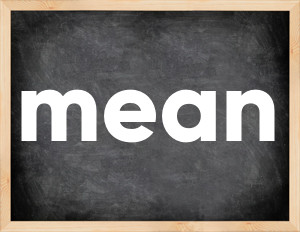# Mean past tenseThe English verb 'mean' is pronounced as [mi:n].
Related to: irregular verbs.
3 forms of verb mean: Infinitive (mean), Past Simple - (meant), Past Participle - (meant).

## Here are the past tense forms of the verb mean

👉 Forms of verb mean in future and past simple and past participle.
❓ What is the past tense of mean.

## Mean: Past, Present, and Participle Forms

Base Form Past Simple Past Participle
mean [mi:n]

meant [mɛnt]

meant [mɛnt]

## What are the 2nd and 3rd forms of the verb mean?

🎓 What are the past simple, future simple, present perfect, past perfect, and future perfect forms of the base form (infinitive) 'mean'?

### Learn the three forms of the English verb 'mean'

• the first form (V1) is 'mean' used in present simple and future simple tenses.
• the second form (V2) is 'meant' used in past simple tense.
• the third form (V3) is 'meant' used in present perfect and past perfect tenses.

## What are the past tense and past participle of mean?

The past tense and past participle of mean are: mean in past simple is meant, and past participle is meant.

### What is the past tense of mean?

The past tense of the verb "mean" is "meant", and the past participle is "meant".

### Verb Tenses

Past simple — mean in past simple meant (V2).
Future simple — mean in future simple is mean (will + V1).
Present Perfect — mean in present perfect tense is meant (have/has + V3).
Past Perfect — mean in past perfect tense is meant (had + V3).

### mean regular or irregular verb?

👉 Is 'mean' a regular or irregular verb? The verb 'mean' is irregular verb.

## Examples of Verb mean in Sentences

•   She meant the whole world to him (Past Simple)
•   The prize meant a lot to him, not just \$1800 (Present Simple)
•   I meant the red dress, not the pink one with patterns (Past Simple)
•   I didn't mean to hurt you, I was scared myself (Past Simple)
•   Nobody wanted to know what it meant (Past Simple)
•   I will explain you later what it means (Future Simple)
•   Staying chaste meant a lot only couple of centuries ago (Past Simple)
•   If only I knew English to understand what it means! (Present Simple)
•   A gift from you means a lot to me (Present Simple)
•   I'm really angry and I mean it (Present Simple)

Along with mean, words are popular shut and climb.

Verbs by letter: , , , , , , , , , , , , , , , , , , , , , , , , .